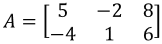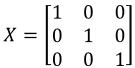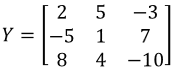# Properties of scalar multiplication

### Properties of scalar multiplication

In this lesson, we will look at the properties of matrix scalar multiplication. These properties include the dimension property for scalar multiplication, associative property, and distributive property. The dimension property states that multiplying a scalar with a matrix (call it A) will give another matrix that has the same dimensions as A. For the associative property, changing the order in which you multiple the matrices has no effect on the final computation. The distributive property states that a scalar can be distributed to the addition or subtraction of matrices. The addition or subtraction of scalars can also be distributed to a matrix. Lastly, we will learn that there is a multiplication property for zero matrices. This property states that multiplying a zero scalar with a matrix will result in a zero matrix. In addition, any scalar multiplied by a zero matrix will result in a zero matrix.

#### Lessons

Note
Let $X,Y$ be matrices with equal dimensions, and $c$ and $d$ be scalars. Then we have the following scalar multiplication properties:

Dimension property for scalar multiplication
The matrix $c \cdot X$ has the same dimensions as $X$.
Associative property
$c \cdot X=X \cdot c$
$c \cdot (dX)=d \cdot (cX)=(dc)X$
Distributive property
$c(X+Y)=cX+cY$
$(c+d)X=cX+dX$

There are also some scalar multiplication properties with the zero matrix

Multiplication property for the zero matrix
$0 \cdot X=O$
$c \cdot O=O$
• Introduction
Properties of matrix scalar multiplication

• 1.
Let. Show that the following is true:
a)
$0 \cdot A=O$

b)
$1 \cdot A=A$

• 2.
You are given that,, $c=5$ and $d=3$. Show that:
a)
$c(X+Y)=cX+cY$

b)
$(c+d)Y=cY+dY$

c)
$(c+d)(X+Y)=c(X+Y)+d(X+Y)$